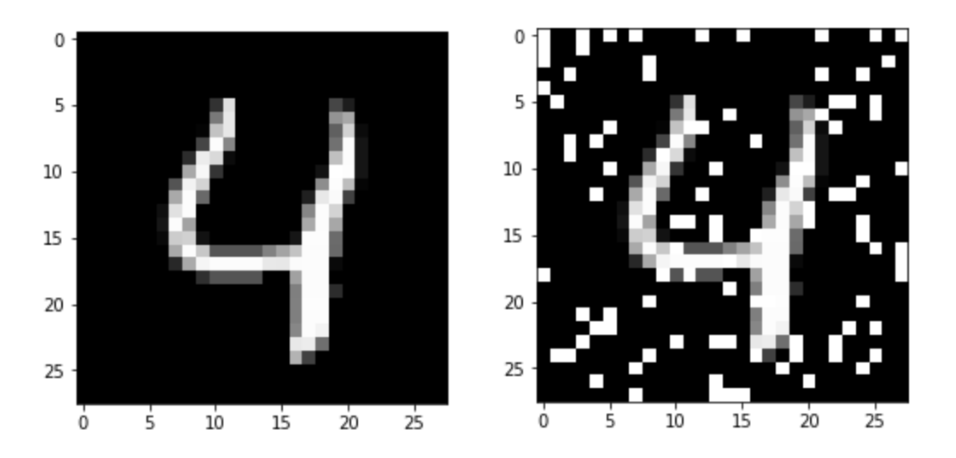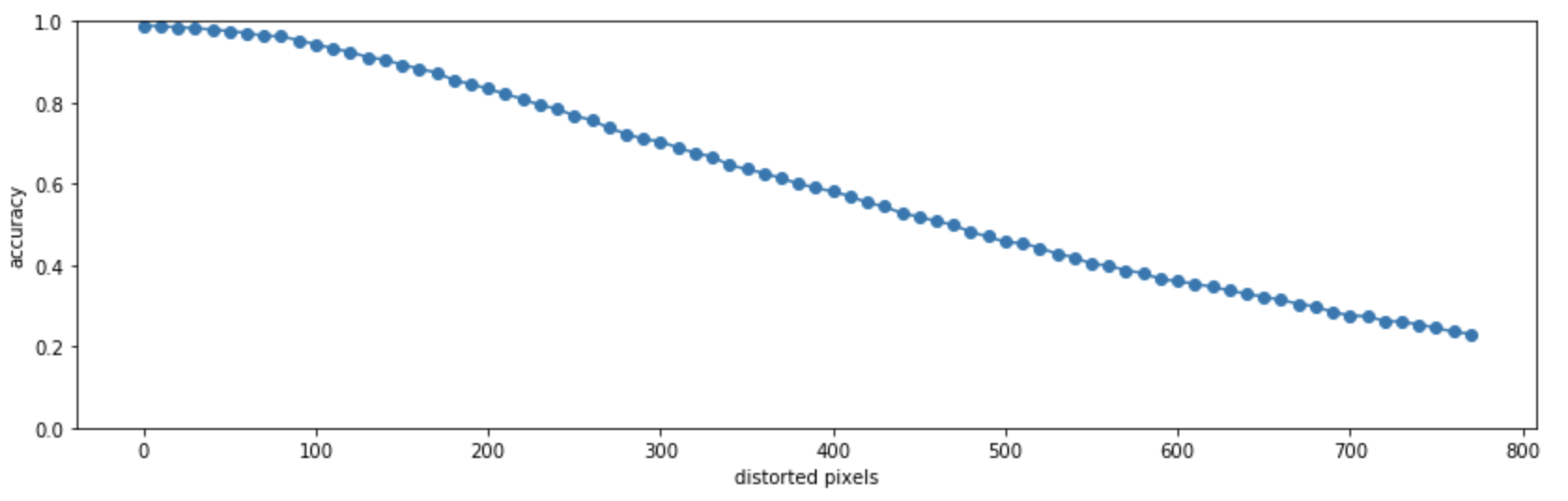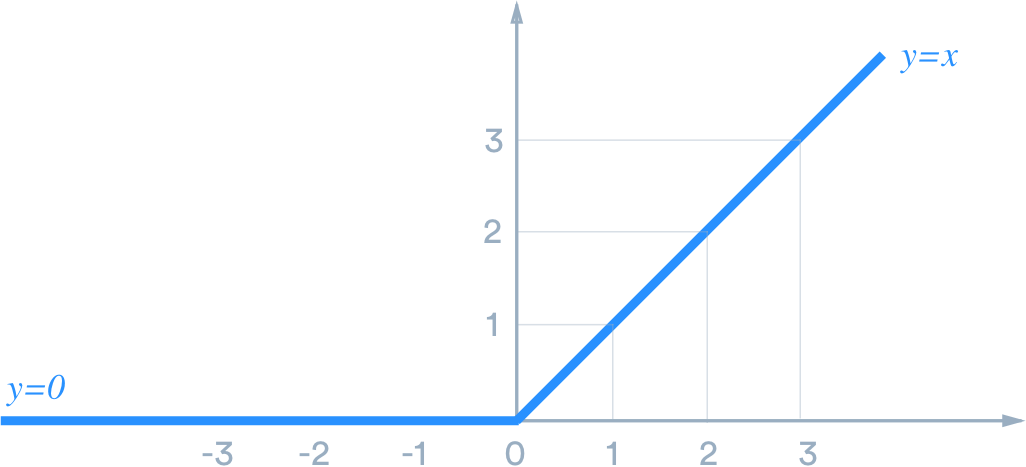MNIST pixel attacks with Pytorch

Posted on Sat 01 June 2019 in Machine Learning

Introduction

It’s easy to build a CNN that does well on MNIST digit classification. How easy is it to break it, to distort the images and cause the model to misclassify? The post was inspired by 2 papers, both are worth reading:

For the experiments, I’m using the ~99% accurate CNN that I’ve trained in the previous MNIST post. The ipython notebook is up on Github.

Random noise

The first and simplest thing I tried is adding random noise. I wanted to see how random noise leads to classification errors, ie. reduced accuracy. Pytorch’s transformer framework made these experiments easy: I just had to add one Lambda() to the transformers chain:

def distort(x, num_pixels=1, value=1.0):
for _ in range(num_pixels):
x[int(random()*28)][int(random()*28)] = value
return x

def plot_accuracies(distorted_pixels, accuracies):
clear_output()
plt.figure(figsize=(14, 4))
plt.xlabel('distorted pixels')
plt.ylabel('accuracy')
plt.ylim((0, 1))
plt.plot(distorted_pixels, accuracies, marker='o')
plt.show()

distorted_pixels = []
accuracies = []
for i in range(0, 20):
datasets.MNIST(
'../data',
train=False,
transform=transforms.Compose([
transforms.ToTensor(),
transforms.Lambda(lambda x: distort(x, num_pixels=i)),
# the above line was added compared to the original model
transforms.Normalize((0.1307,), (0.3081,)),
])
),
batch_size=1000,
shuffle=True)
actuals, predictions = test_label_predictions(model, device, my_test_loader)
distorted_pixels.append(i)
accuracies.append(accuracy_score(actuals, predictions))
plot_accuracies(distorted_pixels, accuracies)

Note that the transforms.Lambda() was added after the transforms.ToTensor(), so at this point the image is a 28x28 tensor of floats between 0 and 1, and before the transforms.Normalize(). For reference, this is what a distorted image looks like (fifth test image in MNIST, a digit 4, original and with 100 pixels distorted):The result shows the decline in accuracy, as a function of how many pixels are randomly distorted on the test image:The accuracy degradation is pretty linear. I was surprised by this, I was expecting the model to perform well up to a certain distortion, and then break down (more inclined inverse S-curve). Note that I set the pixels randomly, so at high counts it’s possible that a pixel is “set twice”, so the actual number of distorted pixels is lower.

One-pixel attacks

Based on the above, breaking the model by setting one-pixel shouldn’t be easy. But in the random noise example, we were setting pixels randomly; is it possible to find a special vulnerable pixel and break the classification? When looking for a one-pixel vulnerability, there are 3 parameters, x and y, and the pixel value. Here I will only play with x and y, I will set the pixel value to 1.0.

Unlike in the article referenced in the intro, here I’m doing a brute-force attack. Two outer loops go through the pixel location, and each time it runs the test images through the model, and counts how many images are misclassified. Note that without any distortion the model achieves 98.9% accuracy, so out of 10,000 test images there are 110 that are misclassified even without distortion. These 110 are always subtracted, they are not counted.

The code:

def set_pixel(x, i, j, value=1.0):
x[i][j] = value
return x

def misclassified_images(actuals, predictions):
vuls = []
for i in range(len(actuals)):
if actuals[i] != predictions[i]:
vuls.append(i)
return set(vuls)

datasets.MNIST(
'../data',
train=False,
transform=transforms.Compose([
transforms.ToTensor(),
transforms.Normalize((0.1307,), (0.3081,)),
])
),
batch_size=1000,
shuffle=False)
actuals, predictions = test_label_predictions(model, device, my_test_loader)
base_misclassifieds = misclassified_images(actuals, predictions)

vulnerable_images = set([])
for i in range(28):
for j in range(28):
datasets.MNIST(
'../data',
train=False,
transform=transforms.Compose([
transforms.ToTensor(),
transforms.Lambda(lambda x: set_pixel(x, i, j)),
transforms.Normalize((0.1307,), (0.3081,)),
])
),
batch_size=1000,
shuffle=False)
actuals, predictions = test_label_predictions(model, device, my_test_loader)
misc = misclassified_images(actuals, predictions)
actual_vulnerables = misc.difference(base_misclassifieds)
print('(%s, %s) vulnerables: %s' % (i, j, actual_vulnerables))
for a in actual_vulnerables:
print('--> %s is %s, classified as %s' % (a, actuals[a], predictions[a]))
vulnerable_images.update(actual_vulnerables)

print(len(base_misclassifieds), len(vulnerable_images))

In the end, it finds only 69 (out of 10,000) that are vulnerable. At most locations, it finds vulnerabilities, but it’s always the same images. For example, image #3906 is very vulnerable, changing the (0,8) pixel gets this 1 classified as a 3:This seems to go against the first cited article’s finding, where ~75% of CIFAR-10 images are found to have a one-pixel vulnerability. Possible explanations:

• classifying digits is simpler than image categories, so MNIST is less vulnerable
• playing around with the pixel values would yield more vulnerabilities

Out-of-bounds one-pixel attacks

Finally, I played around with setting out-of-bounds values, ie. what if I numerically set the pixel value to be greather than 1.0. Let’s repeat the distortion experiment, but set values to 5.0 instead of 1.0. At 20 distorted pixels the accuracy already drops to ~50%:I attibute this to the relu() activation, which cuts off at the low end, but not on the high-end, so these out-of-bounds values are able to travel through the deep layers and throw off the model:Intuitively, neural nets find blobs in high-dimensional space and assign it to a label, and by doing this we move the image away from the blob along one dimension.